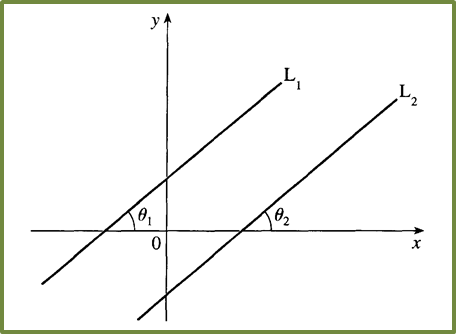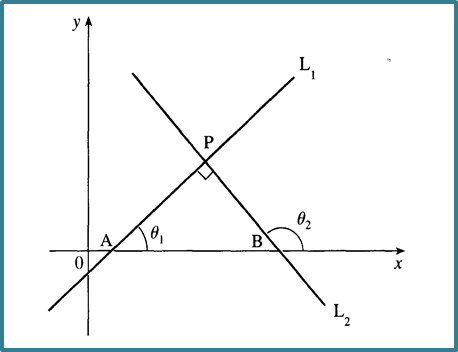# 6.5 Parallel Lines and Perpendicular Lines

6.5 Parallel Lines and Perpendicular Lines

(A) Parallel Lines
1. If two straight lines are parallel, they have same gradient.In the above diagram, if straight line Lis parallel to straight line L2, gradient of L= gradient of L2
$\overline{){m}_{1}={m}_{2}}$

Example 1:
Given that the equation of a straight line parallel to x + 8y= 40 and passes through the point A(2, 3k) and B (-6, 4k2), find the values of k.

Solution:

(B) Perpendicular Lines
1. If two lines are perpendicular to each other, the product of their gradients is 1.In the above diagram, if straight line Lis perpendicular to straight line L2,
$\overline{){m}_{1}×{m}_{2}=-1}$
$\begin{array}{l}{m}_{PQ}=\frac{2-4}{4-\left(-2\right)}=-\frac{1}{3}\\ {m}_{RS}=\frac{6-\left(-3\right)}{2-\left(-1\right)}=3\\ \left({m}_{PQ}\right)\left({m}_{RS}\right)=\left(-\frac{1}{3}\right)\left(3\right)=-1\end{array}$